# Analysis: Global Longwave Radiation Emissions

Longwave (LW) thermal radiation emissions from a planet’s surface play an important role in planetary heat flows and the global energy balance. The overall energy balance operates at the level of global totals or, equivalently, global-average values.

With that in mind, let us obtain useful formulas for the average of the radiative flux of both surface longwave radiation emissions (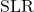) and outgoing longwave radiation emissions (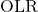).

## Average Surface Longwave Radiation Emissions (SLR)

The Stefan-Boltzmann (SB) law tells us that the surface temperature,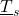, and the radiative flux of surface LW thermal radiation emissions,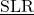, are related by:

(1)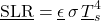where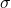is the Stefan-Boltzmann constant and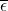is the emissivity, a value between 0 and 1, typically fairly close to 1, which depends the the characteristics of the material doing the emitting. The underline notation is used to indicate quantities that depend on location and time.

The surface temperature varies in time, and also depending on where one is on the planet. Emissivity varies also, mostly by location, but sometimes over time.

Climate data is usually averaged over some time window. For purposes of looking at the global energy balance, it is also useful to average over location, i.e, latitude and longitude.

In this analysis, I will make use of definitions and identities defined on the page Averages and Correlations. The symbol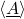denotes the global time-average of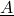;is the weighted average ofwith respect to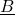; and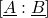is the multiplicative correlation factor forand. Note that working with averages is equivalent to working with Sums and Correlations.

Averaging the Stefan-Boltzmann equation and doing some trivial rewriting leads to:

(2)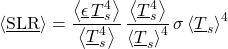This can be expressed simply as:

(3)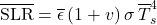where

(4)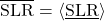(5)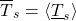(6)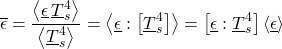(7)The overbar notation is used to denote quantities suitable for using in global time-averaged equations.

The quantities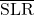and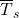are simple area-weighted averages. Some other global quantities are defined as weighed averages.

In particular, the quantityis a global weightedaverage emissivity; this differs from the area-averaged emissivity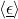by a correlation factor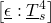. Becauseis a weighted average of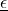, it follows thatis constrained to be between the minimum and maximum values of. So, we know that the factorwill never adjust the emissivity to be outside this range.

The quantitydeviates from 0 only to the extent that the planetary surface temperature is non-uniform. It can be proven mathematically (via an application of Holder’s inequality) that it is always true that:

(8)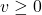So, the factorboosts the emissions (i.e.,) for a given average temperature, relative to the emissions that would be produced if temperature didn’t vary. So, I’ll refer toas the temperature-variation emissions boost factor (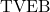). This factor reflects the fact that a system in which the temperature varies from the average value emits more than a system with a uniform temperature. This is true whether those variations occur over time or between locations around the globe. (In practice, for Earth, temperature variations over latitude are the main contributor to this factor.)

Note that while the factorwill never adjust the emissivity to be outside the range 0 to 1, the “boost factor”could potentially lead to an effective emissivity,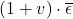greater than 1. (That happens for the Moon, but not for Earth at present.)

What we have achieved is a transformation of the Stefan-Boltzmann equation from an equation that applies at an individual location and time to a similar equation which applies to suitably averaged values,,, and. In the process, it has been necessary to introduce a new parameter,, which effectively boosts emissions. This reflects the fact that a system in which the temperature varies from the average value emits more than a system with a uniform temperature. This is true whether those variations occur over time or between locations around the globe. (On Earth, temperature variations between the tropics and the polar regions are the primary type of temperature variation contributing to.

The same result can expressed in terms of sums. The total energy of thermal radiation emitted by the surface of the globe over some period of time,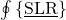, is given by:

(9)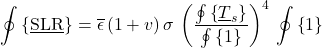The factor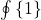is simply the surface area of the planet times the duration of the period over which emissions are summed.

## Average Outgoing Longwave Radiation Emissions (OLR)

The Greenhouse effect is defined quantitatively as:

(10)Alternatively, one can work with the dimensionless normalized Greenhouse effect:

(11)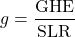The quantity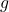can be thought of either as the normalized Greenhouse effect or as the longwave effective absorptance (LEA) of the atmosphere. The standard definition of absorptance is the fraction of radiation power incident on an absorbing medium that is absorbed. In comparison, the LEA,, is the the fraction of longwave thermal radiation power emitted by the surface that, as viewed from space, is absorbed by the atmosphere without having been replaced by an equivalent amount of power from atmospheric longwave emissions. So, LEA is like absorptance, but it accounts for both absorption and emissions.

Note that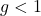. For a planet with an atmosphere transparent to the surface’s thermal radiation (with nothing in it to absorb, emit, reflect, or scatter that radiation) the value ofwould necessarily be 0. Thus, ifis non-zero (as it the case on Earth), this is possible only because of the presence of materials in the atmosphere that interact with longwave radiation.

Given the definition of, it follows that:

(12)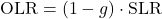(13)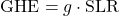This equations could be interpreted either locally or globally, since each of the quantities is well-defined in either context.

Let’s interpret the above equation foras a local equation and average it, yielding:

(14)This may be written in terms of global quantities as:

(15)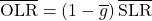(16)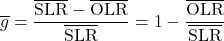where:

(17)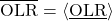(18)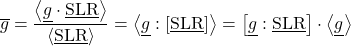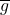is the global weighted-average normalized Greenhouse effect, which differs from the global area-weighted average of the local normalized Greenhouse effect,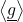, by the multiplicative correlation factor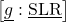. Becauseis a weighted average of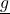, it is constrained to be between the minimum and maximum values of.

Combining this with our prior equation for, t follows that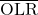is given by:

(19)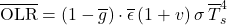Equivalently, the same result can expressed in terms of sums. The total energy of thermal radiation emitted to space over the surface of the globe over some period of time,, is given by:

(20)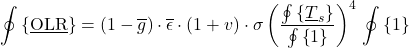## Conclusion

This analysis provides formulas for the global average radiative flux of longwave radiation thermal emissions from the surface,, and the global average radiative flux of longwave radiation thermal emissions outgoing from the top of the atmosphere,These are exact results, involving no approximations. The results rely only on the definitions of the terms involved. Beyond those definitions, the resulting formulas are the exact result of mathematical transformations and tautologies.

The Stefan-Boltzmann law for thermal emissions is the only principle of physics that has been used. The S-B law is a fundamental law of physics with applies to all matter in local thermal equilibrium (LTE), a condition which is easily met for materials on Earth’s surface.1Though not relevant to the current result, LTE also holds throughout Earth’s atmosphere up to an altitude of around 37 km. This derivation constitutes a simple, tautologically-correct transformation of the S-B law, with full mathematical rigor.